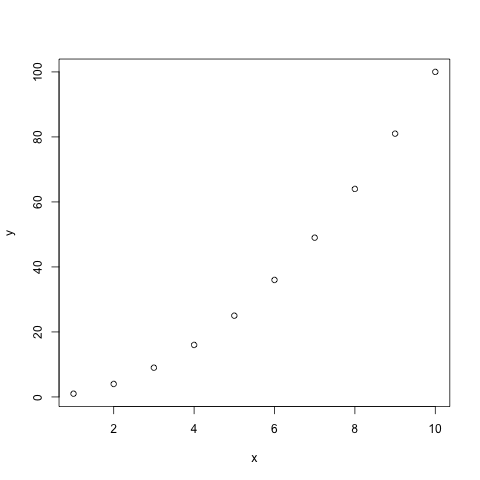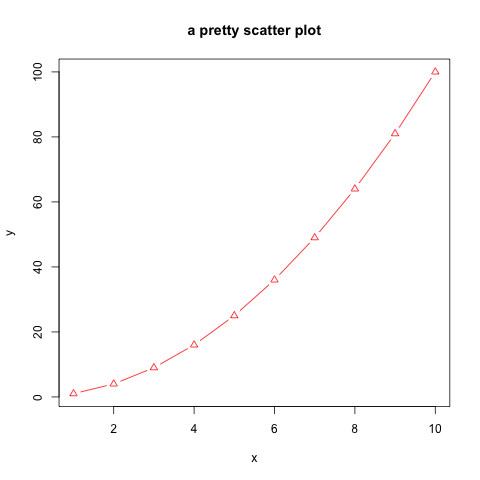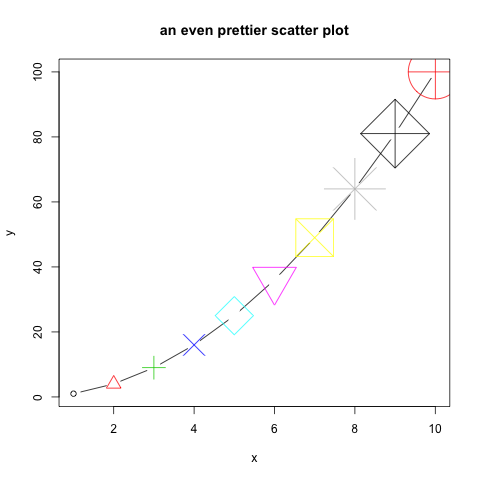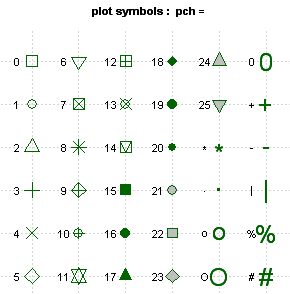## 16.1 Scatter plots

A scatter plot has points that show the relationship between two sets of data.

• Simple scatter plot
``````# Create 2 vectors
x <- 1:10
y <- x^2

# Plot x against y
plot(x, y)``````Note that if one vector only is given as an input, it will be plotted against the indices of each element

• col: color
• pch: type of point
• type: “l” for line, “p” for point, “b” for both point and line
• main: title of the plot
``````plot(x, y,
col="red",
pch=2,
type="b",
main="a pretty scatter plot")``````• You can play a bit:
``````plot(x, y,
col=1:10,
pch=1:10,
cex=1:10,
type="b",
main="an even prettier scatter plot")``````#### Different type of points that you can use:• Color codes 1 to 8 are taken from the palette() function and respectively code for: “black”, “red”, “green3”, “blue”, “cyan”, “magenta”, “yellow”, “gray”.
``````# see the 8-color palette:
palette()``````
• There is a larger set of build-in colors that you can use:
``````# see all 657 possible build-in colors:
colors()

# looking for blue only? You can pick from 66 blueish options:
grep("blue", colors(), value=TRUE)``````

You can also find them here.

• change the default palette with one of your choice:
``palette(grep("blue", colors(), value=TRUE))``
• change the palette back to default
``palette("default")``